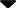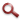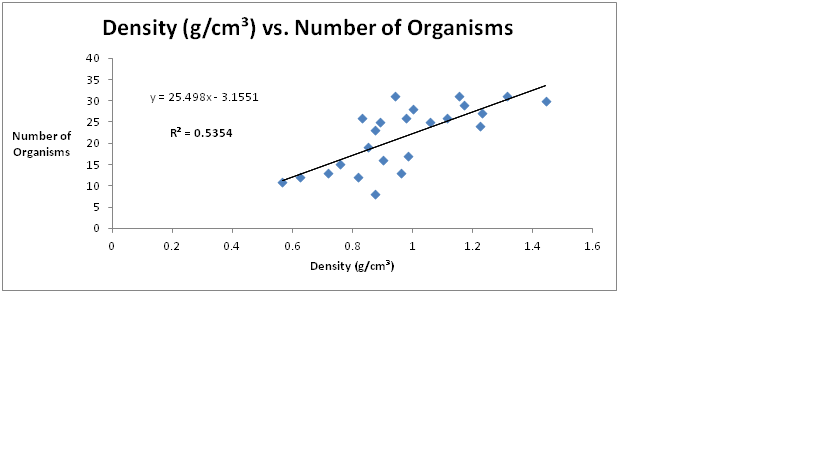Site WebYou are here:

## Student Project FiguresFigure 12 Density (g/cm³) vs. Number Organisms. (N=24) Data points (not jittered) represent number of organisms counted on each ascidian. Line represents linearity and R² value Pearson Correlation Coefficient. A two-sided t-test revealed significant (p<0.05) effect of density on number of organisms (t = 6.7048, df = 47, p-value = P<0.0001). Figure 11 Figure 12